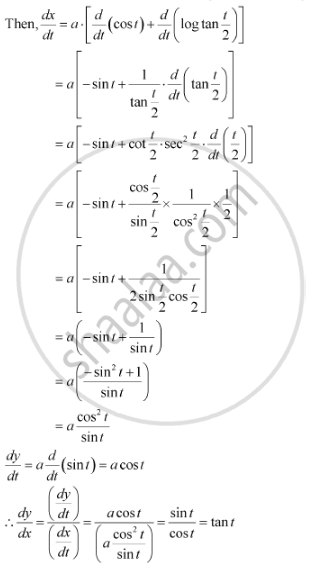Share

# If X And Y Are Connected Parametrically by the Equation, Without Eliminating the Parameter, Find Dy/Dx X = A(Cos T + Log Tan T/2), Y = a Sin T - CBSE (Commerce) Class 12 - Mathematics

ConceptDerivatives of Functions in Parametric Forms

#### Question

If x and y are connected parametrically by the equation, without eliminating the parameter, find dy/dx

x = a(cos t + log tan  t/2), y =  a sin t

#### Solution

The given equations are x = a(cos t + log tan  t/2), y =  a sin tIs there an error in this question or solution?

#### APPEARS IN

NCERT Solution for Mathematics Textbook for Class 12 (2018 to Current)
Chapter 5: Continuity and Differentiability
Q: 8 | Page no. 181
Solution If X And Y Are Connected Parametrically by the Equation, Without Eliminating the Parameter, Find Dy/Dx X = A(Cos T + Log Tan T/2), Y = a Sin T Concept: Derivatives of Functions in Parametric Forms.
S